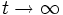# Constant-scalar curvature metric

This article defines a property that makes sense for a Riemannian metric over a differential manifold

This is the property of the following curvature being constant: scalar curvature

## Definition

### Symbol-free definition

A Riemannian metric on a differential manifold is said to be a constant-scalar curvature metric if the scalar curvature at all points is equal.

### Definition with symbols

Fill this in later

## Relation with other properties

### Stronger properties

The following properties of Riemannian metrics are stronger than the property of being a constant-scalar curvature metric:

## Flows

### Yamabe flow

The Riemannian metrics with this property are precisely the stationary points for this flow: Yamabe flow

The Yamabe conjecture states that every conformal class of Riemannian metrics contains a constant-scalar curvature metric. The Yamabe flow is intended to help answer this conjecture positively. The Yamabe flow has, for its stationary points, the metrics of constant scalar curvature. Further, the Yamabe flow preserves the conformal class of the Riemannian metric.

The idea is to start with an arbitrary member of the conformal class, evolve it using the Yamabe flow, and take the limit as$t \to \infty$.